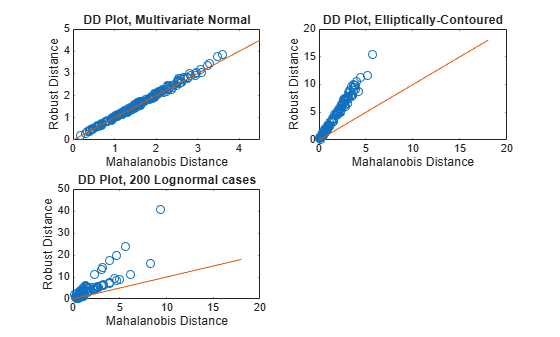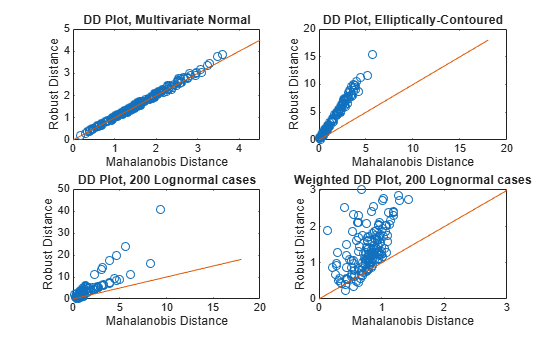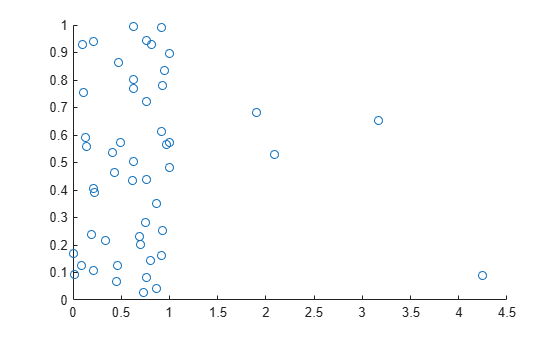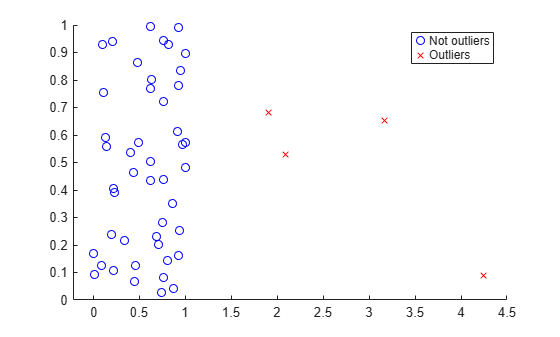# robustcov

Robust multivariate covariance and mean estimate

## Syntax

``sig = robustcov(x)``
``````[sig,mu] = robustcov(x)``````
``````[sig,mu,mah] = robustcov(x)``````
``````[sig,mu,mah,outliers] = robustcov(x)``````
``````[sig,mu,mah,outliers,s] = robustcov(x)``````
``[___] = robustcov(x,Name,Value)``

## Description

example

````sig = robustcov(x)` returns the robust covariance estimate `sig` of the multivariate data contained in `x`.```
``````[sig,mu] = robustcov(x)``` also returns an estimate of the robust Minimum Covariance Determinant (MCD) mean, `mu`.```
``````[sig,mu,mah] = robustcov(x)``` also returns the robust distances `mah`, computed as the Mahalanobis distances of the observations using the robust estimates of the mean and covariance.```
``````[sig,mu,mah,outliers] = robustcov(x)``` also returns the indices of the observations retained as outliers in the sample data, `outliers`.```

example

``````[sig,mu,mah,outliers,s] = robustcov(x)``` also returns a structure `s` that contains information about the estimate.```

example

````[___] = robustcov(x,Name,Value)` returns any of the arguments shown in the previous syntaxes, using additional options specified by one or more `Name,Value` pair arguments. For example, you can specify which robust estimator to use or the start method to use for the attractors.```

## Examples

collapse all

Use a Gaussian copula to generate random data points from a bivariate distribution.

```rng default rho = [1,0.05;0.05,1]; u = copularnd('Gaussian',rho,50);```

Modify 5 randomly selected observations to be outliers.

```noise = randperm(50,5); u(noise,1) = u(noise,1)*5;```

Calculate the robust covariance matrices using the three available methods: Fast-MCD, Orthogonalized Gnanadesikan-Kettenring (OGK), and Olive-Hawkins.

```[Sfmcd, Mfmcd, dfmcd, Outfmcd] = robustcov(u); [Sogk, Mogk, dogk, Outogk] = robustcov(u,'Method','ogk'); [Soh, Moh, doh, Outoh] = robustcov(u,'Method','olivehawkins');```

Calculate the classical distance values for the sample data using the Mahalanobis measure.

```d_classical = pdist2(u, mean(u),'mahal'); p = size(u,2); chi2quantile = sqrt(chi2inv(0.975,p));```

Create DD Plots for each robust covariance calculation method.

```tiledlayout(2,2) nexttile plot(d_classical, dfmcd, 'o') line([chi2quantile, chi2quantile], [0, 30], 'color', 'r') line([0, 6], [chi2quantile, chi2quantile], 'color', 'r') hold on plot(d_classical(Outfmcd), dfmcd(Outfmcd), 'r+') xlabel('Mahalanobis Distance') ylabel('Robust Distance') title('DD Plot, FMCD method') hold off nexttile plot(d_classical, dogk, 'o') line([chi2quantile, chi2quantile], [0, 30], 'color', 'r') line([0, 6], [chi2quantile, chi2quantile], 'color', 'r') hold on plot(d_classical(Outogk), dogk(Outogk), 'r+') xlabel('Mahalanobis Distance') ylabel('Robust Distance') title('DD Plot, OGK method') hold off nexttile plot(d_classical, doh, 'o') line([chi2quantile, chi2quantile], [0, 30], 'color', 'r') line([0, 6], [chi2quantile, chi2quantile], 'color', 'r') hold on plot(d_classical(Outoh), doh(Outoh), 'r+') xlabel('Mahalanobis Distance') ylabel('Robust Distance') title('DD Plot, Olive-Hawkins method') hold off```In a DD plot, the data points tend to cluster in a straight line that passes through the origin. Points that are far removed from this line are generally considered outliers. In each of the previous plots, the red '+' symbol indicates the data points that `robustcov` considers to be outliers.

This example shows how to use `robustcov` to evaluate sample data for multivariate normal or other elliptically-contoured (EC) distributions.

Generate random sample data from a multivariate normal distribution. Calculate the Mahalanobis distances for the robust covariance estimates (using the Olive-Hawkins method) and the classical covariance estimates.

```rng('default') x1 = mvnrnd(zeros(1,3),eye(3),200); [~, ~, d1] = robustcov(x1,'Method','olivehawkins'); d_classical1 = pdist2(x1,mean(x1),'mahalanobis');```

Generate random sample data from an elliptically-contoured (EC) distribution. Calculate the Mahalanobis distances for the robust covariance estimates (using the Olive-Hawkins method) and the classical covariance estimates.

```mu1 = [0 0 0]; sig1 = eye(3); mu2 = [0 0 0]; sig2 = 25*eye(3); x2 = [mvnrnd(mu1,sig1,120);mvnrnd(mu2,sig2,80)]; [~, ~, d2] = robustcov(x2, 'Method','olivehawkins'); d_classical2 = pdist2(x2, mean(x2), 'mahalanobis');```

Generate random sample data from a multivariate lognormal distribution, which is neither multivariate normal or elliptically-contoured. Calculate the Mahalanobis distances for the robust covariance estimates (using the Olive-Hawkins method) and the classical covariance estimates.

```x3 = exp(x1); [~, ~, d3] = robustcov(x3, 'Method','olivehawkins'); d_classical3 = pdist2(x3, mean(x3), 'mahalanobis');```

Create a D-D Plot for each of the three sets of sample data to compare.

```figure subplot(2,2,1) plot(d_classical1,d1, 'o') line([0 4.5], [0, 4.5]) xlabel('Mahalanobis Distance') ylabel('Robust Distance') title('DD Plot, Multivariate Normal') subplot(2,2,2) plot(d_classical2, d2, 'o') line([0 18], [0, 18]) xlabel('Mahalanobis Distance') ylabel('Robust Distance') title('DD Plot, Elliptically-Contoured') subplot(2,2,3) plot(d_classical3, d3, 'o') line([0 18], [0, 18]) xlabel('Mahalanobis Distance') ylabel('Robust Distance') title('DD Plot, 200 Lognormal cases')```For data with a multivariate normal distribution (as shown in the upper left), the plotted points follow a straight, 45-degree line extending from the origin. For data with an elliptically-contoured distribution (as shown in the upper right), the plotted points follow a straight line, but are not at a 45-degree angle to the origin. For the lognormal distribution (as shown in the lower left), the plotted points do not follow a straight line.

It is difficult to identify any pattern in the lognormal distribution plot because most of the points are in the lower left of the plot. Use a weighted DD plot to magnify this corner and reveal features that are obscured when large robust distances exist.

```d3_weighted = d3(d3 < sqrt(chi2inv(0.975,3))); d_classical_weighted = d_classical3(d3 < sqrt(chi2inv(0.975,3)));```

Add a fourth subplot to the figure to show the results of the weighting process on the lognormally distributed data.

```subplot(2,2,4) plot(d_classical_weighted, d3_weighted, 'o') line([0 3], [0, 3]) xlabel('Mahalanobis Distance') ylabel('Robust Distance') title('Weighted DD Plot, 200 Lognormal cases')```The scale on this plot indicates that it represents a magnified view of the original DD plot for the lognormal data. This view more clearly shows the lack of pattern to the plot, which indicates that the data is neither multivariate normal nor elliptically contoured.

Use a Gaussian copula to generate random data points from a bivariate distribution.

```rng default rho = [1,0.05;0.05,1]; u = copularnd('Gaussian',rho,50);```

Modify 5 randomly selected observations to be outliers.

```noise = randperm(50,5); u(noise,1) = u(noise,1)*5;```

Visualize the bivariate data using a scatter plot.

```figure scatter(u(:,1),u(:,2))```Most of the data points appear on the left side of the plot. However, some of the data points appear further to the right. These points are possible outliers that could affect the covariance matrix calculation.

Compare the classical and robust covariance matrices.

`c = cov(u) `
```c = 2×2 0.5523 0.0000 0.0000 0.0913 ```
`rc = robustcov(u)`
```rc = 2×2 0.1117 0.0364 0.0364 0.1695 ```

The classical and robust covariance matrices differ because the outliers present in the sample data influence the results.

Identify and plot the data points that `robustcov` considers outliers.

```[sig,mu,mah,outliers] = robustcov(u); figure gscatter(u(:,1),u(:,2),outliers,'br','ox') legend({'Not outliers','Outliers'})````robustcov` identifies the data points on the right side of the plot as potential outliers, and treats them accordingly when calculating the robust covariance matrix.

## Input Arguments

collapse all

Sample data used to estimate the robust covariance matrix, specified as a matrix of numeric values. `x` is an n-by-p matrix where each row is an observation and each column is a variable.

`robustcov` removes any rows with missing predictor values when calculating the robust covariance matrix.

Data Types: `single` | `double`

### Name-Value Arguments

Specify optional pairs of arguments as `Name1=Value1,...,NameN=ValueN`, where `Name` is the argument name and `Value` is the corresponding value. Name-value arguments must appear after other arguments, but the order of the pairs does not matter.

Before R2021a, use commas to separate each name and value, and enclose `Name` in quotes.

Example: `'Method','ogk','NumOGKIterations',1` specifies the robust estimator as the Orthogonalized Gnanadesikan-Kettenring method and sets the number of orthogonalization iterations to 1.

For All Estimators

collapse all

Robust estimator, specified as one of the following.

NameValue
`'fmcd'`FAST-MCD (Minimum Covariance Determinant) method
`'ogk'`Orthogonalized Gnanadesikan-Kettenring (OGK) estimate
`'olivehawkins'`Concentration algorithm techniques, a family of fast, consistent and highly outlier-resistant methods

Example: `'Method','ogk'`

For the FMCD and OliveHawkins Methods Only

collapse all

Outlier fraction, specified as the comma-separated pair consisting of `'OutlierFraction'` and a numeric value in the range [0,0.5]. The value 1 – OutlierFraction specifies the fraction of observations over which to minimize the covariance determinant.

The algorithm chooses a subsample of size h = ceiling(n + p + 1) / 2), where n is the number of observations and p is the number of dimensions. `OutlierFraction` is the value for which the maximum possible breakdown is achieved, and controls the size of the subsets h over which the covariance determinant is minimized. The algorithm then chooses h to approximately equal (1 – OutlierFraction) × n observations per subset.

Example: `'OutlierFraction',0.25`

Data Types: `single` | `double`

Number of trials, specified as the comma-separated pair consisting of `'NumTrials'` and a positive integer value.

If `'Method'` is `'fmcd'`, then `NumTrials` is the number of random subsamples of size (p + 1) drawn from the sample data as starting points in the algorithm. p is the number of dimensions in the sample data. In this case, the default value for `NumTrials` is 500.

If `'Method'` is `'olivehawkins'`, then `NumTrials` is the number of trial fits, or attractors, to be used. In this case, the default value for `NumTrials` is 2. This option is only useful for non-deterministic starts.

Example: `'NumTrials',300`

Data Types: `single` | `double`

For the FMCD Method Only

collapse all

Flag to apply small-sample correction factor, specified as the comma-separated pair consisting of `'BiasCorrection'` and either `1` or `0`. A `1` value indicates that `robustcov` corrects for bias in the covariance estimate for small samples. A `0` value indicates that `robustcov` does not apply this correction.

Example: `'BiasCorrection',0`

Data Types: `logical`

For the OGK Method Only

collapse all

Number of orthogonalization iterations, specified as the comma-separated pair consisting of `'NumOGKIterations'` and a positive integer value. Generally, this value is set to 1 or 2, and further steps are unlikely to improve the estimation.

Example: `'NumIter',1`

Data Types: `single` | `double`

Function for computing univariate robust estimates, specified as the comma-separated pair consisting of `'UnivariateEstimator'` and one of the following.

NameValue
`'tauscale'`Use the “tau-scale” estimate of Yohai and Zamar, which is a truncated standard deviation and a weighted mean.
`'qn'`Use the Qn scale estimate of Croux and Rousseeuw.

Example: `'UnivariateEstimator','qn'`

For the OliveHawkins Method Only

collapse all

Method for reweighting in the efficiency step, specified as the comma-separated pair consisting of `'ReweightingMethod'` and one of the following.

NameValue
`'rfch'`Uses two reweighting steps. This is a standard method of reweighting to improve efficiency.
`'rmvn'`Reweighted multivariate normal. Uses two reweighting steps that can be useful for estimating the true covariance matrix under a variety of outlier configurations when the clean data are multivariate normal.

Example: `'ReweightingMethod','rmvn'`

Number of concentration steps, specified as the comma-separated pair consisting of `'NumConcentrationSteps'` and a positive integer value.

Example: `'NumConcentrationSteps',8`

Data Types: `single` | `double`

Start method for each attractor, specified as the comma-separated pair consisting of `'Start'` and one of the following.

NameValue
`'classical'`Use the classical estimator as the start. This is the DGK attractor which, used on its own, is known as the DGK estimator.
`'medianball'`Use the Median Ball as the start. The Median Ball is `(med(x),eye(p))`. So 50% of cases furthest in Euclidean distance from the sample median are trimmed for computing the MB start. This is the MB attractor which, used on its own, is known as the MB estimator.
`'elemental'`The attractor is generated by concentration where the start is a randomly selected elemental start: the classical estimator applied to a randomly selected “elemental set” of p + 1 cases. This “elemental” attractor is computationally efficient, but suffers from theoretical drawbacks, as it is inconsistent and zero breakdown.

By default, the attractor is chosen as follows: If one of the attractors is `'medianball'`, then any attractor whose location estimate has greater Euclidean distance from `median(X)` than half the data (in other words, is outside the median ball) is not used. Then the final attractor is chosen based on the MCD criterion.

You can also specify a function handle for a function that returns two output arguments used for computing the initial location and scatter estimates..

You can also specify a cell array containing any combination of the options given in the previous table and function handles. The number of attractors used is equal to the length of the cell array. This option allows more control over the algorithm and the ability to specify a custom number of attractors and starts.

Example: `'StartMethod','medianball'`

## Output Arguments

collapse all

Robust covariance matrix estimates, returned as a p-by-p numeric matrix. p is the number of predictors contained in the sample data.

Robust mean estimates, returned as a 1-by-p array of numeric values. p is the number of predictors contained in the sample data.

Robust Mahalanobis distances, returned as a 1-by-n array of numeric values. `robustcov` removes any rows of `x` that contain missing data, so the number of rows of `mah` might be smaller than the number of rows in `x`.

Indices of observations retained as outliers in the sample data `x`, returned as a 1-by-n array of logical values. A `0` value indicates that the observation is not an outlier. A `1` value indicates that the observation is an outlier.

`robustcov` removes any rows of `x` that contain missing data, so the number of rows of `outliers` might be smaller than the number of rows in `x`.

Structure containing estimate information, returned as a structure.

collapse all

### Mahalanobis Distance

The Mahalanobis distance is a measure between a sample point and a distribution.

The Mahalanobis distance from a vector x to a distribution with mean μ and covariance Σ is

`$d=\sqrt{\left(x-\mu \right){\sum }^{-1}\left(x-\mu \right)\text{'}}.$`

The distance represents how far x is from the mean in number of standard deviations.

`robustcov` returns the robust Mahalanobis distances (`mah`) from observations in `x` to the distribution with mean `mu` and covariance `sig`.

## Algorithms

collapse all

### Minimum Covariance Determinant Estimate

Minimum covariance determinant (MCD) is the fastest estimator of multivariate location and scatter that is both consistent and robust. However, an exact evaluation of the MCD is impractical because it is computationally expensive to evaluate all possible subsets of the sample data. `robustcov` uses the FAST-MCD method to implement MCD 

The FAST-MCD method selects h observations out of n (where n/2 < hn) whose classical covariance matrix has the lowest possible determinant. The MCD mean is the mean of the h selected observations.

The MCD covariance is the covariance matrix of the h selected points, multiplied by a consistency factor to obtain consistency at the multivariate normal distribution, and by a correction factor to correct for bias at small sample sizes.

Orthogonalized Gnanadesikan-Kettenring (OGK) estimate is a positive definite estimate of the scatter starting from the Gnanadesikan and Kettering (GK) estimator, a pairwise robust scatter matrix that may be non-positive definite . The estimate uses a form of principal components called an orthogonalization iteration on the pairwise scatter matrix, replacing its eigenvalues, which could be negative, with robust variances. This procedure can be iterated for improved results, and convergence is usually obtained after 2 or 3 iterations.

### Olive Hawkins Estimate

The Olive-Hawkins estimate uses the “concentration algorithm” techniques proposed by Olive and Hawkins. This is a family of fast, consistent, and highly outlier-resistant methods. The estimate is a robust root n-consistent estimator of covariance for elliptically contoured distributions with fourth moments. This estimate is obtained by first generating trial estimates, or starts, and then using the concentration technique from each trial fit to obtain attractors.

Suppose (T0j,C0j) is a start, then at the next iteration the classical mean and covariance estimators are computed from the approximately n / 2 cases (where n is the number of observations) with the smallest Mahalanobis distances based on the estimates from the previous iteration. This iteration can be continued for a fixed number of steps k, with the estimate at the last step, k, being the attractor. The final estimate is chosen based on a given criterion.

By default, two attractors are used. The first attractor is the Devlin-Gnanadesikan-Kettering (DGK) attractor, where the start used is the classical estimator. The second attractor is the Median Ball (MB) attractor, where the start used is `(median(x),eye(p))`, in other words the half set of data closest to `median(x)` in Euclidean distance. The MB attractor is used if the location estimator of the DGK attractor is outside of the median ball, and the attractor with the smallest determinant is used otherwise. The final mean estimate is the mean estimate of the chosen attractor, and the final covariance estimate is the covariance estimate of the chosen attractor, multiplied by a scaling factor to make the estimate consistent at the normal distribution.

 Maronna, R. and Zamar, R.H.. “Robust estimates of location and dispersion for high dimensional datasets.” Technometrics, Vol. 50, 2002.

 Pison, S. Van Aelst and G. Willems. “Small Sample Corrections for LTS and MCD.” Metrika, Vol. 55, 2002.

 Rousseeuw, P.J. and Van Driessen, K. “A fast algorithm for the minimum covariance determinant estimator.” Technometrics, Vol. 41, 1999.

 Olive, D.J. “A resistant estimator of multivariate location and dispersion.” Computational Statistics and Data Analysis, Vol. 46, pp. 99–102, 2004.